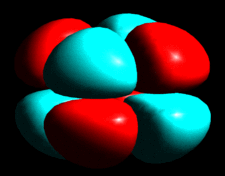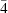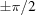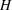# issue contentsFOUNDATIONSADVANCES
ISSN: 2053-2733

# January 2001 issueCover illustration: Graphical representation of density modification due to third-order terms b113 = -b223 (point group) in the Gram-Charlier series expansion of a Gaussian atomic probability density function. Courtesy of W. F. Kuhs.

## research papers

### The crystal problem for polytypes

The question of crystalline ground states for polytypes is considered, using reasoning based on the symmetries of the modular layers. It is found that polytypes in the SiC family must have crystalline ground states; but this conclusion does not hold for the other families studied (CdI2, GaSe, micas and kaolins).

### A geometric centroid principle and its application

A fundamental geometric principle opens up non-classical qualitative ways of investigating orientational disorder.

### Structural units of cuprates in natural Cu-oxysalts

Copper oxysalt minerals are considered as the salts of hypothetical copper acids with characteristic Cu-containing structural units. Magnetic susceptibility data for a series of minerals were analyzed from the viewpoint of the developed extended structural classification scheme for cuprates.

### The method of joint probability distribution functions applied to the one-wavelength anomalous-scattering (OAS) case

The joint probability distribution method is applied to define phases for the one-wavelength anomalous-scattering techniques.

### A probabilistic approach to space-group determination from powder diffraction data

The determination of the space-group symmetry of a crystal from powder diffraction data is placed on a quantitative basis using an algorithm based upon probability theory.

### Estimation of individual phases from the four-phase structure invariants in the single isomorphous replacement case

A method is presented that can be used to estimate some individual phases with special values of 0, π orusing the SIR data in macromolecular structure determination.

### Structure refinement of the silicon carbide polytypes 4H and 6H: unambiguous determination of the refinement parameters

`Quasiforbidden' reflections are utilized for an unambiguous determination of structure refinement parameters of the silicon carbide polytypes 4and 6. The determination was accomplished by a comparison of measured and calculated Renninger scans close to three-beam nodes.

### Forbidden X-ray wavefields of three-beam Bragg reflections from thick crystals

A detailed analysis of a three-beam diffraction dispersion surface is performed to study the forbidden wavefields of thick-crystal reflections. It is demonstrated that Bragg-case three-beam diffraction from thick crystals can be regarded as either a four-mode diffraction process for Bragg–Laue geometry or a two-mode process for Bragg–Bragg geometry.

### Wavefunctions derived from experiment. I. Motivation and theory

The concept of extracting wavefunctions from experimental data is critically examined and past efforts are reviewed. A general and systematically improvable method for fitting a wavefunction to experimental data is also proposed.

### Wavefunctions derived from experiment. II. A wavefunction for oxalic acid dihydrate

A wavefunction has been calculated for the oxalic acid dihydrate molecule using accurate low-temperature X-ray charge-density data. Derived properties from the constrained Hartree–Fock wavefunction fragments are also presented.

### Diffuse X-ray scattering from benzil, C14H10O2: analysis via automatic refinement of a Monte Carlo model

A recently developed method for fitting a Monte Carlo computer simulation model to observed single-crystal diffuse X-ray scattering data has been used to study the diffuse scattering in benzil. The analysis has shown that the diffuse lines, which feature so prominently in the observed diffraction patterns, are due to strong longitudinal displacement correlations transmitted from molecule to molecule via a network of contacts involving hydrogen bonding.

## short communications

### The invariant cubic rod (cylinder) packings: symmetries and coordinates

There are six homogeneous cubic packings of non-intersecting rods (cylinders) with the positions of the axes fixed by symmetry (invariant). Their symmetries and coordinates are given.

### On the symmetry of 4- to 11-hedra

A table giving the statistics of the visible symmetry point groups for all non-isomorphic 4- to 8-hedra and simple 9- to 11-hedra is presented.

### Coset and double-coset decompositions of the magnetic point groups

Mathematical properties of crystallographic magnetic point groups are tabulated.

### A pseudoinverse for Frank's formula

The Moore–Penrose generalized inverse to Frank's formula for grain boundary dislocation content is derived using a simple vector approach.